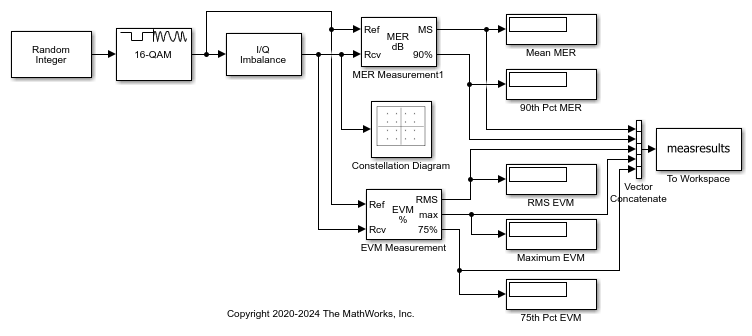EVM and MER Measurements Using Simulink

This example shows how to compute error vector magnitude (EVM) and modulation error rate (MER) measurements using Simulink® blocks.

Explore Model

The model adds I/Q imbalance to a 16-QAM signal. The EVM Measurement and MER Measurement blocks perform EVM and MER measurements, respectively, on the impaired signal. The Constellation Diagram block displays the impaired signal constellation and signal quality measurements. The signal quality measurements displayed in the constellation diagram window approximate the measurements reported by the EVM Measurement and MER Measurement blocks.In this model, the I/Q Imbalance block sets the amplitude imbalance to 1 dB, the phase imbalance to 15 degrees, and the DC offset to 0. The MER Measurement block is configured to output the mean MER, and the 90th percentile MER. The EVM Measurement block is configured to output the RMS EVM (normalized to the average reference signal power), the maximum EVM, and the 75th percentile EVM.

Run Model and Display Measurements

Run the model to compute the EVM and MER measurements and to display the constellation diagram.

Average MER is 16.9 dB.
90 percentile MER is 14.0 dB.
RMS EVM is 14.3%.
Maximum EVM is 20.7%.
75 percentile EVM is 17.5%.Change the I/Q Imbalance block setting for the amplitude imbalance to 2 dB. Because the modulation accuracy decreases when the impairment value increases, the reported average MER decreases and the reported average EVM increases. Run the model again to see the degraded EVM and MER metrics.Average MER is 13.2 dB.
90 percentile MER is 10.2 dB.
RMS EVM is 21.8%.
Maximum EVM is 31.2%.
75 percentile EVM is 26.8%.